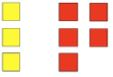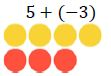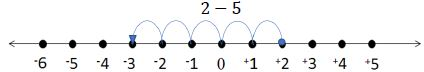# MA.6.NSO.4.1Export Print
Apply and extend previous understandings of operations with whole numbers to add and subtract integers with procedural fluency.

### Clarifications

Clarification 1: Instruction begins with the use of manipulatives, models and number lines working towards becoming procedurally fluent by the end of grade 6.
Clarification 2:
Instruction focuses on the inverse relationship between the operations of addition and subtraction. If p and q are integers, then p-q=p+(-q) and p+q=p-(-q).
General Information
Subject Area: Mathematics (B.E.S.T.)
Strand: Number Sense and Operations
Status: State Board Approved

## Benchmark Instructional Guide

• Equal Sign
• Equation
• Integers
• Number Line
• Whole Number

### Vertical Alignment

Previous Benchmarks

http://flbt5.floridaearlylearning.com/standards.html

Next Benchmarks

### Purpose and Instructional Strategies

In elementary grades, students added and subtracted whole numbers using models, including number lines and counters. These skills are extended in grade 6 to compare and perform operations with integers with procedural fluency as well as write and solve single-step equations using integers. In grade 7, students work with all operations with rational numbers in the form of expressions, equations and inequalities.
• It can be beneficial for students to start learning to add and subtract integers using real world contexts such as, the movement of a football on a football field, above and below sea level, temperature; refer to Appendix A for more situations. From the real-world context, develop a conceptual model to represent the situation. From the situation, students can describe the relationship mathematically as an expression.
• Explore opportunities with students to discover patterns or rules instead of being directly instructed and expected to memorize them. It is not expected or recommended that students start by generating equivalent expressions mathematically (MTR.2.1, MTR.5.1, MTR.7.1).
• Use models to help students identify patterns for adding and subtracting integers. Instruction includes opportunities to model adding and subtracting integers using tools such as algebra tiles, two-color counters and number lines. Students should relate the model to the expression (MTR.5.1).
• Algebra Tiles
+3 + (−5)• Two-Color Counters
5 + (−3)• For example, if a student is evaluating 5 + (−3) using two-colored counters, a student can think, “I start with 5 yellows and 3 reds. A positive and a negative together make a zero-sum pair. So if I have 5 positive yellows and 3 negative reds, 3 of the paired chips will have a sum of zero, and I will have 2 yellow positives remaining, so my answer is 2.”
• Number Lines• For example, if a student is evaluating 2−5 using a number line, a student can think, “I start at positive 2 and move 5 in the negative direction (to the left). I am now at –3.” Students can also use the commutative property to change the order to (−5) + 2. The student can see that if they start at −5 and move 2 in the positive direction (right), landing on –3.
• When using algebra tiles or two-color counters, group the positive and negative values to create zero-sum pairs, then the remaining tiles or counters show the resulting value.
• When combining integers on a number line, a negative symbol (“–”) denotes the direction that will be traveled. If two negative symbols “−(−)” or subtracting a negative number is present, the operators are showing to switch to the opposite direction of movement twice; the first negative symbol (−) indicates moving to the left on the number line, whereas the second negative symbol (−) indicates moving the opposite direction, or to the right, so it is the same as adding the second addend.
• Instruction includes understanding that subtracting a positive value is the same as adding a negative value-both move the value left or decrease it on the number line. The commutative property can help students to see the relationship. Subtracting a negative value is the same as adding a positive value, moving the value to the right.
• Procedural fluency is developed with the combination of conceptual understanding and practice. Students should be encouraged to think through why a sum or difference would result in a positive or negative value instead of encouraged to memorize a set of rules (MTR.3.1).
• The commutative property of addition allows for individuals to rearrange the order of the terms they are adding regardless if they are positive or negative terms (MTR.3.1).
• For example, 6−3 can be rewritten as 6 + (−3) in order to the commutative property to see that this is equivalent to (−3) + 6.
• Use real-world contexts that model opposite relationships, like borrowing/earning money or falling/climbing, to help students conceptually reason through why a solution would be positive or negative (MTR.6.1).
• Instruction includes connecting understanding of the magnitudes of numbers to the absolute value of a number.
• Students should develop fluency with and without the use of a calculator when performing operations with integers.
• Instruction includes the use of models to demonstrate that the commutative and associative properties does not apply to subtraction. Demonstrate the correct use of the commutative and associative properties of addition by rewriting a subtraction expression as an addition expression before applying either property.
• For example, 6−3 ≠ 3−6, but 6−3 = 6+(−3) and 6−3 = (−3)+6.
• For example, (3−6) + 5 ≠ 3−(6+5), but (3−6)+5 = 3+(−6)+5 and (3−6)+5 = 3+(−6+5).

### Common Misconceptions or Errors

• Some students may misunderstand when adding and subtracting integers, the sign of the “bigger number” is kept.
• For example, in the expression –8 + 4, the sum will be negative because –8 is the “bigger number.” Instead, it can be thought of as there are more negatives than positives being combined, so the result will be negative value.
• Students may confuse zero for being a negative number because it is less than a whole. However, 0 is a neutral number; it is neither positive nor negative.
• Students may incorrectly believe that the number with the larger absolute value will always be the minuend (the first term in a subtraction expression) or that the positive number must always be the minuend. This may stem from an overgeneralization of the common pattern in elementary mathematics that “the larger number always goes first in subtraction.”

### Strategies to Support Tiered Instruction

• Teacher provides opportunities for students to utilize two-color counters to represent addition and subtraction of integers. Use repeated addition to develop generalizations for multiplying integers and discuss the difference between generalized rules for addition and generalized rules for multiplication.
• Teacher provides opportunities for students to utilize two-color counters when evaluating the sum or difference of integers. Instruction includes having students write the expression, representing the expression with the two-color counters, finding the sum or difference, and then recording the solution.
• Teacher models identifying zero pairs using manipulatives such as two-color counters.

At 9:00 a.m. the temperature was −5°F. By noon, the temperature had risen 14°F. On a number line, show how to find the temperature at noon. Explain.

Jonah is a novice when it comes to scuba diving. His first dive was 12 feet deep, and his second dive was 3 feet deeper than the first. Describe the depth of Jonah’s second dive. Show your work on a vertical number line.

At 6:00 p.m., the temperature was 11°C. By midnight, the temperature was −3°C.
Part A. Using a visual model, show how to find the difference between the temperatures.
Part B. Write a numerical expression to represent the temperature change from 6:00 p.m. to midnight. Explain.

Write −3 − 6 as an equivalent addition problem. How are −3 − 6 and the addition problem you wrote related? Explain using a visual model.

### Instructional Items

Instructional Item 1
Evaluate –5 − 8 − (−2).

Instructional Item 2
What is the value of the expression 9 + (−12)?

*The strategies, tasks and items included in the B1G-M are examples and should not be considered comprehensive.

## Related Courses

This benchmark is part of these courses.
1200400: Foundational Skills in Mathematics 9-12 (Specifically in versions: 2014 - 2015, 2015 - 2022, 2022 and beyond (current))
1205010: M/J Grade 6 Mathematics (Specifically in versions: 2014 - 2015, 2015 - 2022, 2022 and beyond (current))
1205020: M/J Accelerated Mathematics Grade 6 (Specifically in versions: 2014 - 2015, 2015 - 2020, 2020 - 2022, 2022 and beyond (current))
1204000: M/J Foundational Skills in Mathematics 6-8 (Specifically in versions: 2014 - 2015, 2015 - 2022, 2022 and beyond (current))
7812015: Access M/J Grade 6 Mathematics (Specifically in versions: 2014 - 2015, 2015 - 2018, 2018 - 2022, 2022 and beyond (current))

## Related Access Points

Alternate version of this benchmark for students with significant cognitive disabilities.
MA.6.NSO.4.AP.1: Use tools to add and subtract integers between 50 and −50.

## Related Resources

Vetted resources educators can use to teach the concepts and skills in this benchmark.

## Formative Assessments

Type: Formative Assessment

Finding the Difference:

Students are asked to find the difference between two integers using a number line.

Type: Formative Assessment

Students are asked to rewrite a subtraction problem as an equivalent addition problem and explain the equivalence using a number line.

Type: Formative Assessment

Students are asked to describe an example of additive inverse and demonstrate the concept on a number line.

Type: Formative Assessment

## Lesson Plans

Have You Met Your Match?-Understanding Equivalent Expressions :

In this lesson, students will use the properties of operations to generate and identify equivalent algebraic expressions. Students will apply their prior knowledge of the distributive property and combining like terms to create equivalent algebraic expressions. The hands-on memory "match" game will engage students and support student understanding of algebraic thinking.

Type: Lesson Plan

Order Matters:

Students will analyze a Scratch program and compare its computerized algorithm to the mathematical order of operations, in this lesson plan.

Type: Lesson Plan

What are binary numbers?:

Students will decode equations to explain how base ten numbers are represented using binary numbers.

Type: Lesson Plan

## Original Student Tutorial

Learn how to explain the meaning of additive inverse, identify the additive inverse of a given rational number, and justify your answer on a number line in this original tutorial.

Type: Original Student Tutorial

## MFAS Formative Assessments

Students are asked to describe an example of additive inverse and demonstrate the concept on a number line.

Finding the Difference:

Students are asked to find the difference between two integers using a number line.

Students are asked to rewrite a subtraction problem as an equivalent addition problem and explain the equivalence using a number line.

## Original Student Tutorials Mathematics - Grades 6-8

Learn how to explain the meaning of additive inverse, identify the additive inverse of a given rational number, and justify your answer on a number line in this original tutorial.

## Student Resources

Vetted resources students can use to learn the concepts and skills in this benchmark.